Courses

# Test: Building With Bricks - 2

## 15 Questions MCQ Test Mathematics (Maths) for Class 4 | Test: Building With Bricks - 2

Description
This mock test of Test: Building With Bricks - 2 for Class 4 helps you for every Class 4 entrance exam. This contains 15 Multiple Choice Questions for Class 4 Test: Building With Bricks - 2 (mcq) to study with solutions a complete question bank. The solved questions answers in this Test: Building With Bricks - 2 quiz give you a good mix of easy questions and tough questions. Class 4 students definitely take this Test: Building With Bricks - 2 exercise for a better result in the exam. You can find other Test: Building With Bricks - 2 extra questions, long questions & short questions for Class 4 on EduRev as well by searching above.
QUESTION: 1

### Is the given shape open or closed?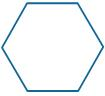Solution:

Look at the given shape.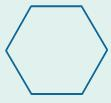Start by tracing this shape. If the start and end points are at the same position, then it is closed. Otherwise, it is open.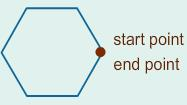Here, we notice that the start and end points are at same positions.
So, the given shape is closed.

QUESTION: 2

### Is the given shape open or closed?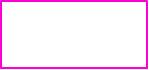Solution:

Look at the given shape.Start by tracing this shape. If the start and end points are at the same position, then it is closed. Otherwise, it is open.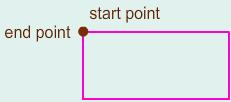Here, we notice that the start and end points are at same position.
So, the given shape is closed.

QUESTION: 3

### Is the given shape open or closed?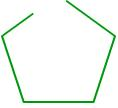Solution:

Look at the given shape.Start by tracing this shape. If the start and end points are at the same position, then it is closed. Otherwise, it is open.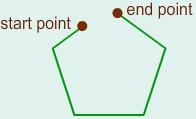Here, we notice that the start and end points are at different positions.
So, the given shape is open.

QUESTION: 4

Which of the following shapes has fewer sides?

Solution:

Sides are the straight lines that make a flat shape.
Let’s consider the given shapes one by one and find the total number of sides for each.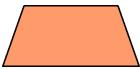This shape has 4 sides.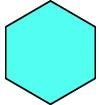This shape has 6 sides.
So, shape A has fewer sides.

QUESTION: 5

Which of the following shapes has 4 corners?

Solution:

A corner is a point where two sides meet.
Let’s consider the given shapes one by one and find the total number of corners for each.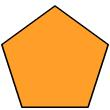This shape has 5 corners.This shape has 4 corners.
So, shape B has 4 corners.

QUESTION: 6

Which of the following shapes has 6 angles?

Solution:

An angle is formed where two sides of a shape meet.
Let’s consider the given shapes one by one and find the total number of angles for each.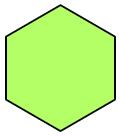This shape has 6 angles.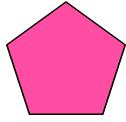This shape has 5 angles.
So, shape A has 6 angles.

QUESTION: 7

Which of the following shapes has 5 corners?

Solution:

A corner is a point where two sides meet.
Let’s consider the given shapes one by one and find the total number of corners for each.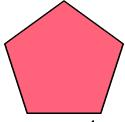This shape has 5 corners.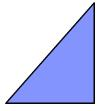This shape has 3 corners.
So, shape A has 5 corners.

QUESTION: 8

Which of the following shapes has 4 angles?

Solution:

An angle is formed where two sides of a shape meet.
Let’s consider the given shapes one by one and find the total number of angles for each.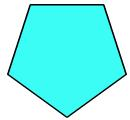This shape has 5 angles.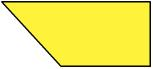This shape has 4 angles.
So, shape B has 4 angles.

QUESTION: 9

Which of the following shapes has 3 angles?

Solution:

An angle is formed where two sides of a shape meet.
Let’s consider the given shapes one by one and find the total number of angles for each.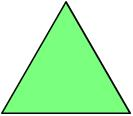This shape has 3 angles.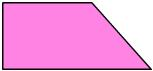This shape has 4 angles.
So, shape A has 3 angles.

QUESTION: 10

Which of the following shapes has fewer sides?

Solution:

Sides are the straight lines that make a flat shape.
Let’s consider the given shapes one by one and find the total number of sides for each.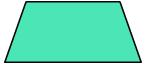This shape has 4 sides.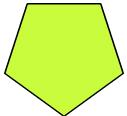This shape has 5 sides.
So, shape A has fewer sides.

QUESTION: 11

Which of the following shapes has fewer sides?

Solution:

Sides are the straight lines that make a flat shape.
Let’s consider the given shapes one by one and find the total number of sides for each.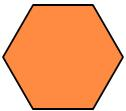This shape has 6 sides.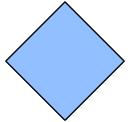This shape has 4 sides.
So, shape B has fewer sides.

QUESTION: 12

Which of the following shapes has 4 sides?

Solution:

Sides are the straight lines that make a flat shape.
Let’s consider the given shapes one by one and find the total number of sides for each.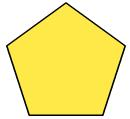This shape has 5 sides.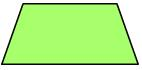This shape has 4 sides.
So, shape B has 4 sides.

QUESTION: 13

Which of the following shapes has 4 corners?

Solution:

A corner is a point where two sides meet.
Let’s consider the given shapes one by one and find the total number of corners for each.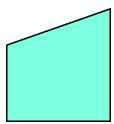This shape has 4 corners.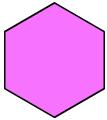This shape has 6 corners.
So, shape A has 4 corners.

QUESTION: 14

Which of the following shapes has fewer angles?

Solution:

An angle is formed where two sides of a shape meet.
Let’s consider the given shapes one by one and find the total number of angles for each.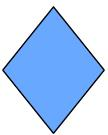This shape has 4 angles.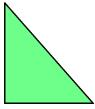This shape has 3 angles.
So, shape B has fewer angles.

QUESTION: 15

Which of the following shapes has fewer angles?

Solution:

An angle is formed where two sides of a shape meet.
Let’s consider the given shapes one by one and find the total number of angles for each.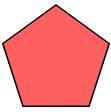This shape has 5 angles.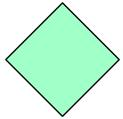This shape has 4 angles.
So, shape B has fewer angles.